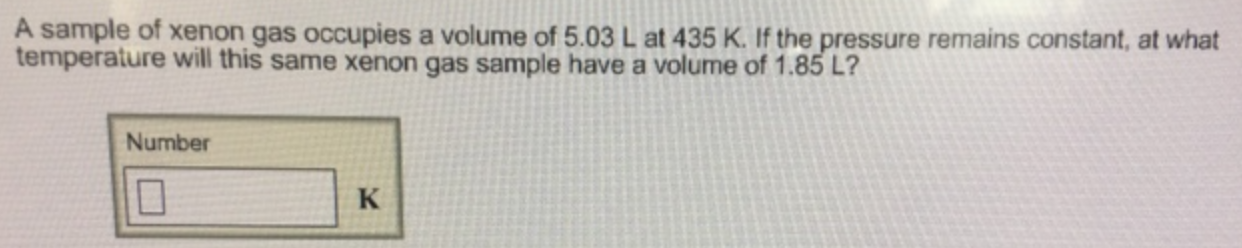Chemistry Practice Problems Chemistry Gas Laws Practice Problems Solution: A sample of xenon gas occupies a volume of 5.03 L ...

# Solution: A sample of xenon gas occupies a volume of 5.03 L at 435 K. If the pressure remains constant, at what temperature will this same xenon gas sample have a volume of 1.85 L?

###### Problem

A sample of xenon gas occupies a volume of 5.03 L at 435 K. If the pressure remains constant, at what temperature will this same xenon gas sample have a volume of 1.85 L?View Complete Written Solution

Chemistry Gas Laws

Chemistry Gas Laws

#### Q. The volume of a gas is 4.58 L, measured at 1.00 atm. What is the pressure of the gas in mmHg if the volume if changed to 8.64 L? (The temperature rema...

Solved • Tue Jul 17 2018 14:03:11 GMT-0400 (EDT)

Chemistry Gas Laws

#### Q. A 18.0 L sample of hydrogen gas has a pressure of 26.0 atm. What volume would this gas occupy at 4.10 atm? Assume ideal behavior.

Solved • Tue Jul 17 2018 12:57:24 GMT-0400 (EDT)

Chemistry Gas Laws

#### Q. A balloon filled with helium has a volume of 11.9 L at 283 K. What volume will the balloon occupy at 251 K?

Solved • Tue Jul 17 2018 12:55:18 GMT-0400 (EDT)

Chemistry Gas Laws

#### Q. What Celsius temperature, T2, is required to change the volume of the gas sample in Part A (T1 = 37°C, V1 = 1.97 x 103 L) to a volume of 3.94 x 103 L?...

Solved • Mon Jul 16 2018 16:13:14 GMT-0400 (EDT)